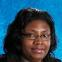Java Games: Flashcards, matching, concentration, and word search.AB
cellIntersection between a row and column
formulaA mathematical equation that is used to calculate spreadsheet values
functionA shortcut math expression for common calculations.
spreadsheetComputer application program used to compute information or predict and estimate future situations.
AVGAverage
*multiply
/divide
=AVG (C3:C13)Example of a function
LabelACCOUNT, DATE, ETC.
SUMFunction for adding group of numbers.
CurrencyTo print dollar signs on a preadsheet, values should be formatted as:Mrs. Snowden Computers, Keyboarding and Careers Acme-Delco Middle School Delco, NC

 This activity was created by a Quia Web subscriber. Learn more about QuiaCreate your own activities